Function Repository Resource:

# PeakExpiratoryFlow

Calculate a person's maximum rate of exhalation

Contributed by: Claudio Lobo Chaib Filho
 ResourceFunction["PeakExpiratoryFlow"][age,height,gender] gives the peak rate of exhalation for a person of a particular age,height and gender. ResourceFunction["PeakExpiratoryFlow"][age,height,gender,prop] gives the property prop.

## Details and Options

Peak expiratory flow is a person's maximum speed of expiration (breathing out air). It measures the airflow through the bronchi and thus the degree of obstruction in the airways.
The age can be given as a Quantity in any unit of time. When a unit is not specified, the interpretation is in years.
The age can be any value between 1 month and 120 years.
The height can be given as a Quantity in any unit of length. When a unit is not specified, the interpretation is in centimeters.
There is no specific range for height. In some cases, where the age and height set are not satisfactory for the function, the "LungCapacity" prop will indicate Missing["NotAvailable"].
Gender (gender) can be given as an Entity or String, e.g. Entity["Gender","Male"] or "Female".
The following properties are supported for prop:
 "PEF" (default) peak expiratory flow value "LungCapacity" estimated lung capacity (volume of the lungs) "Plot" age versus PEF plot "PropertyAssociation" Association containing all properties
"Plot" gives an age (x-axis) versus PEF (y-axis) plot, where the normal region (green) is shown along with the typical curve (black dashed). The warning region is displayed in yellow and the medical emergency region is displayed in red.
The "TimeUnit" option can take any unit of time. By default, the time is given in minutes.
The "VolumeUnit" option can take any unit of volume. By default, the volume is given in liters.

## Examples

### Basic Examples (1)

Calculate the PEF (peak expiratory flow) of a person:

 In:=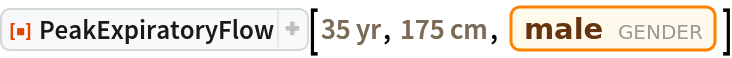Out=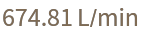### Scope (6)

When the function is used with three arguments, the property given is "PEF":

 In:=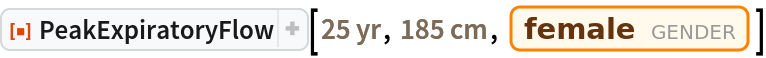Out=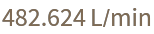In:=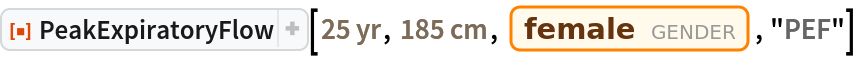Out=If age and height units are not specified, they are interpreted as years and centimeters:

 In:=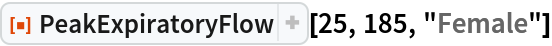Out=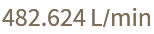Other units of measurement are accepted in the function input, for example, months and feet:

 In:=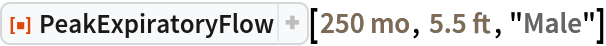Out=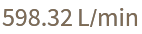Obtain approximate lung capacity with the fourth argument "LungCapacity":

 In:=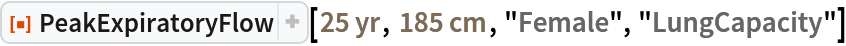Out=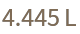Get a plot with the fourth argument "Plot":

 In:=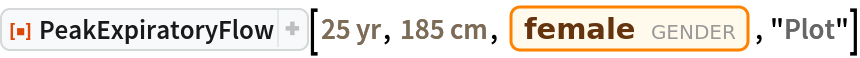Out=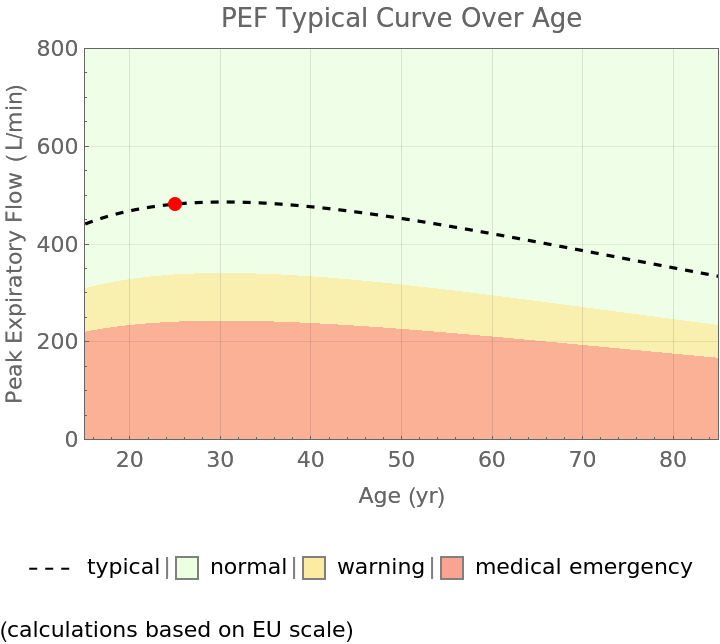Get all forms of output simultaneously as an Association using "PropertyAssociation":

 In:=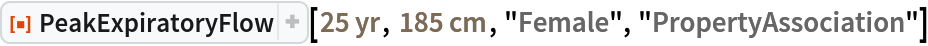Out=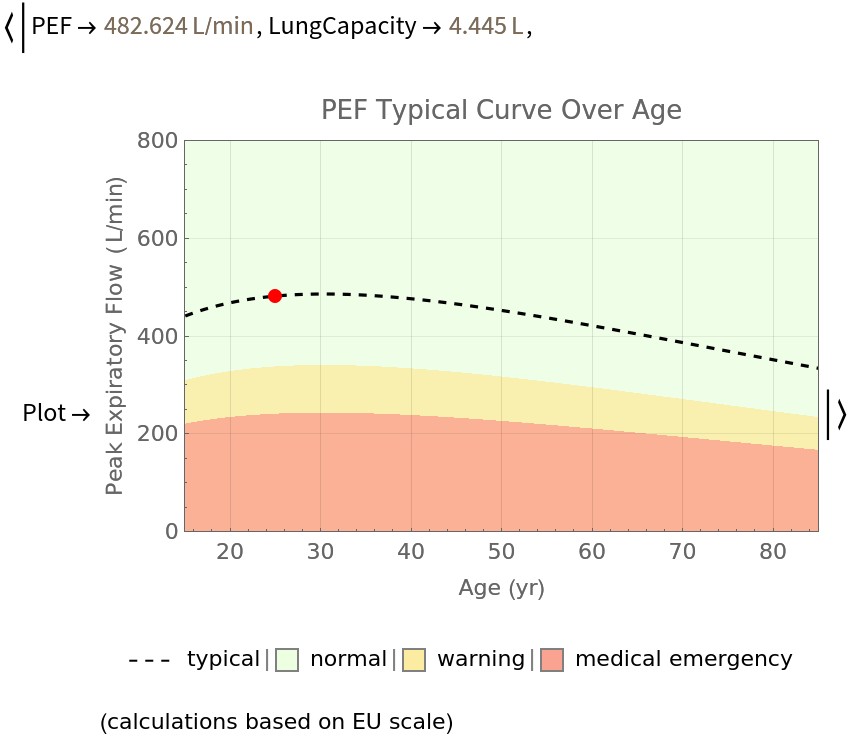### Options (3)

Get a unit of time other than minutes with the option "TimeUnit":

 In:=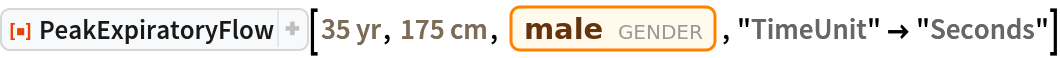Out=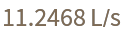Get a unit of volume other than liters with the option "VolumeUnit":

 In:=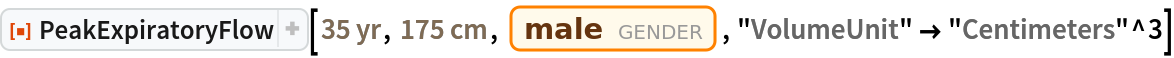Out=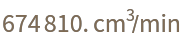All forms of output inherit the specific units chosen by the options, including the plot:

 In:=Out=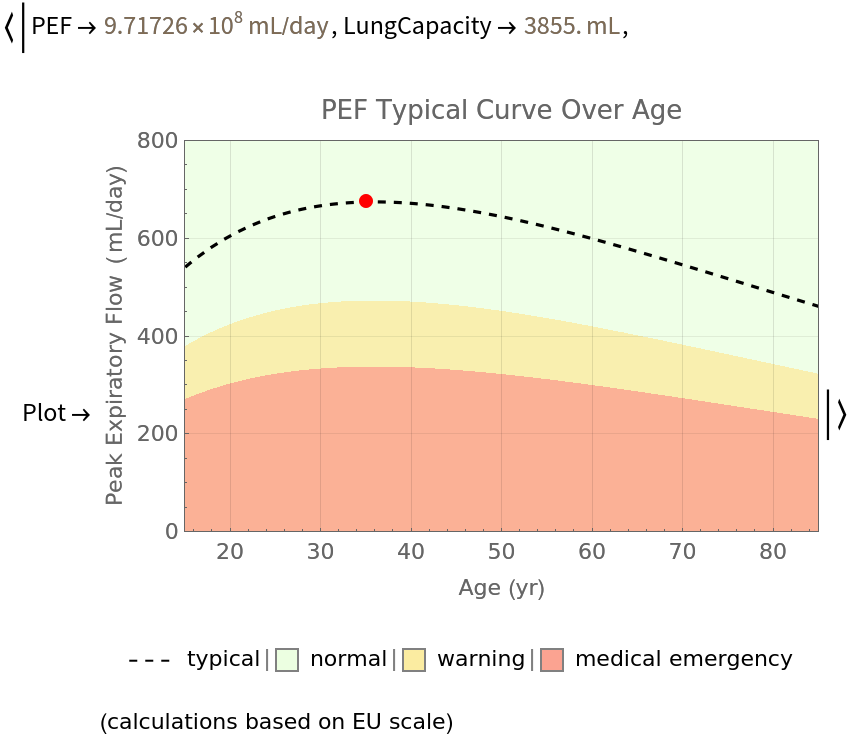### Possible Issues (2)

Even if age is given in a time unit other than years, the x-axis of the plot will be in years:

 In:=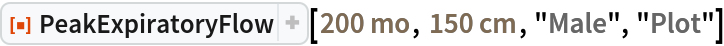Out=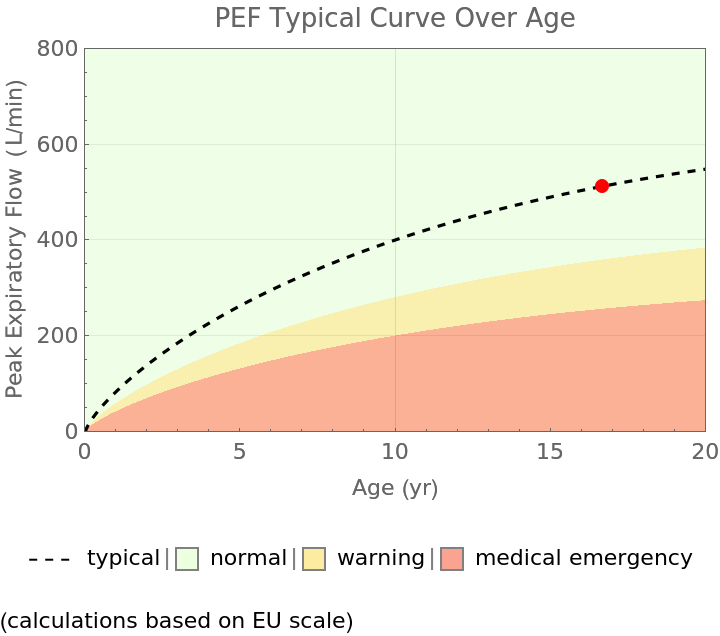There is no specific range for height. In some cases, where the age and height set is not satisfactory for the function, the specific output "LungCapacity" will indicate it is missing:

 In:=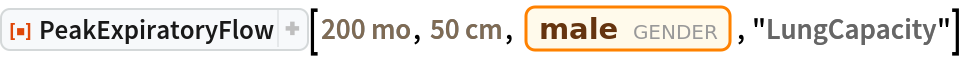Out=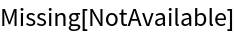## Publisher

Claudio Lobo Chaib Filho

## Version History

• 1.0.0 – 11 November 2020